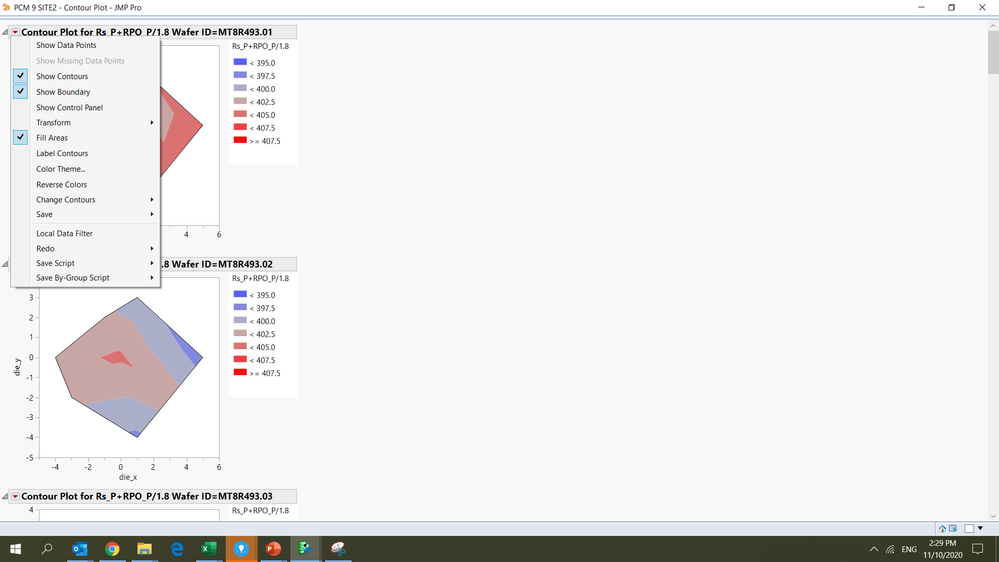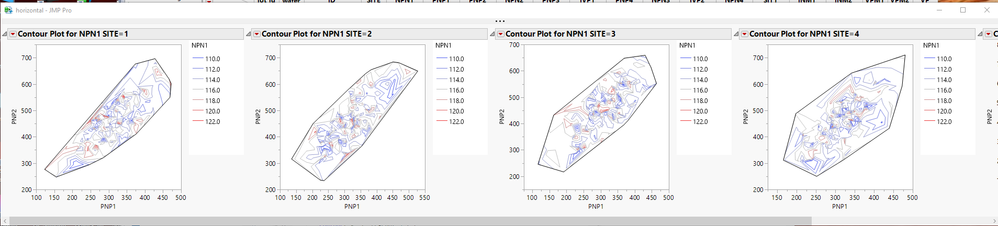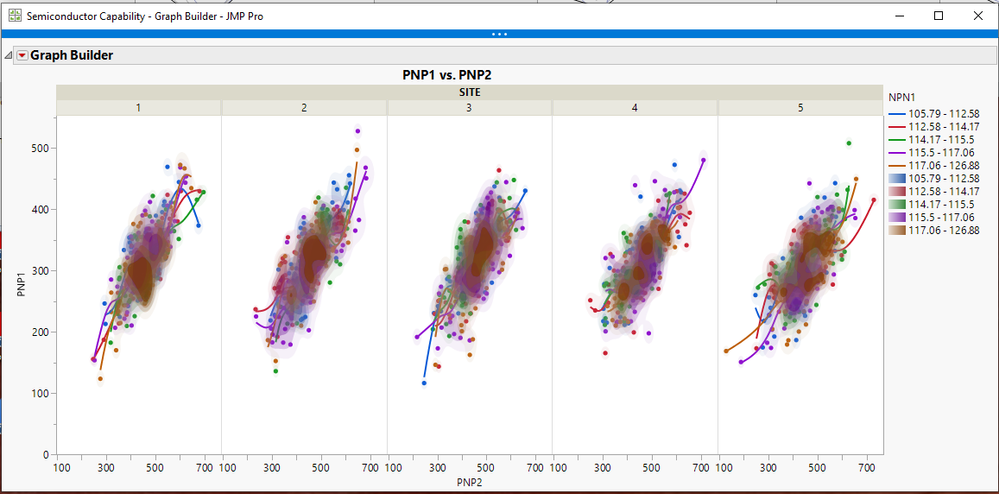Our World Statistics Day conversations have been a great reminder of how much statistics can inform our lives. Do you have an example of how statistics has made a difference in your life? Share your story with the Community!
Choose Language Hide Translation Bar
Highlighted

## Contour Plot arrange by Row

I want to plot contour plot arrange by row group by Wafer ID. By default, the graph will be arrange in columns. Is there a way to display the graph in n rows and n columns that i like?1 ACCEPTED SOLUTION

Accepted Solutions
Highlighted

## Re: Contour Plot arrange by Row

Here are 2 ways to do it.  The first is to place a simple window around the output and specify to use a H List Box() to arrange the different graphs.

The second is to use Graph Builder, and the built in capabilities of that platform.``````Names Default to Here(1);
dt = Open("\$SAMPLE_DATA/semiconductor capability.jmp");

New Window( "horizontal",
H List Box(
Contour Plot(
X( :PNP1, :PNP2 ),
Y( :NPN1 ),
Show Data Points( 0 ),
Fill Areas( 0 ),
Label Contours( 0 ),
Transform( "Range Normalized" ),
Specify Contours(
Min( 110 ),
Max( 122 ),
N( 7 )
),
by( :site )
)
)
);

// or with Graph Builder
Graph Builder(
Size( 1009, 492 ),
Show Control Panel( 0 ),
Variables( X( :PNP2 ), Y( :PNP1 ), Group X( :SITE ), Overlay( :NPN1 ) ),
Elements(
Points( X, Y, Legend( 3 ) ),
Smoother( X, Y, Legend( 4 ) ),
Contour( X, Y, Legend( 5 ) )
)
);``````
Jim
Highlighted

## Re: Contour Plot arrange by Row

Here are 2 ways to do it.  The first is to place a simple window around the output and specify to use a H List Box() to arrange the different graphs.

The second is to use Graph Builder, and the built in capabilities of that platform.``````Names Default to Here(1);
dt = Open("\$SAMPLE_DATA/semiconductor capability.jmp");

New Window( "horizontal",
H List Box(
Contour Plot(
X( :PNP1, :PNP2 ),
Y( :NPN1 ),
Show Data Points( 0 ),
Fill Areas( 0 ),
Label Contours( 0 ),
Transform( "Range Normalized" ),
Specify Contours(
Min( 110 ),
Max( 122 ),
N( 7 )
),
by( :site )
)
)
);

// or with Graph Builder
Graph Builder(
Size( 1009, 492 ),
Show Control Panel( 0 ),
Variables( X( :PNP2 ), Y( :PNP1 ), Group X( :SITE ), Overlay( :NPN1 ) ),
Elements(
Points( X, Y, Legend( 3 ) ),
Smoother( X, Y, Legend( 4 ) ),
Contour( X, Y, Legend( 5 ) )
)
);``````
Jim
Article Labels